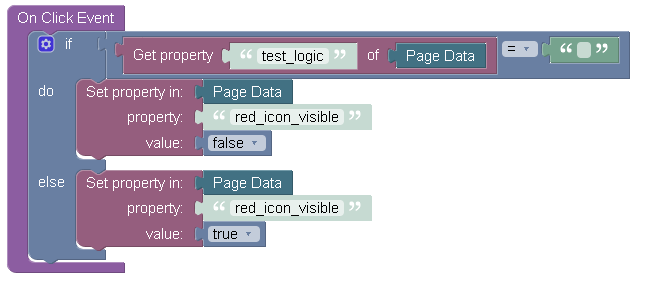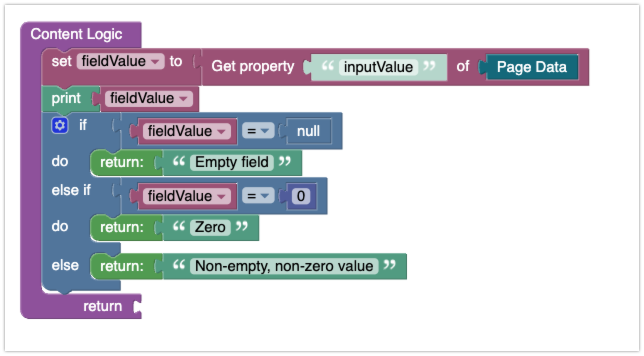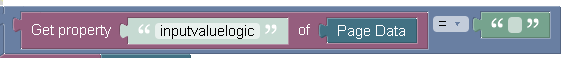# Checking if a property = "" (has no data)

I have an form-input databound to “test_logic” this way
The input is type “number”

I am using the following logic to see if “test_logic” is empty ( IE “” )Looking at the above if statement, I’d expect only one value to hit the “do” section of this if and that’s if test_logic=“”

I have tested and I am seeing a match for “” and for “0” (I know these are not strings because the input type it set to number just using “” to make it clear what I’m looking for )

note that “” does not match null nor is it undefined. My problem is “” is not “0”. I need to test for “” that does not also show the same result for 0.

I ended up converting to a string and then using the length function

which does appear to work. but is this the best solution? and why does “” = 0 when testing in an if statement as shown above?

H

I would start by removing the `On Change Logic` entirely. If you already have data binding, then anything that goes into the field is automatically bound to the property in the `Tabs Content` data model.

If you need to test for an empty string your approach with the sting length is the way to go.

As for `"" = 0`, when the value is empty, JS evaluates it to 0 (or boolean `false`) for conditional expression evaluation purposes.

Regards,
Mark

I think the issue is the input field is set to number (not text) so its not really a string. If a user leaves it blank I need to test for that. if a user enters 0, that is a legitimate number.

if X = “” should not return true if X=0

“” for a number is better represented as undefined or null but not 0

H

Here’s a sample logic that can determine if a numeric input field is empty, contains zero or contains a non-zero value:You can play with a page that implements the logic here:
https://www.backendless.us/api/files/ui-builder/containers/support/index.html?page=empty-string-check

Thanks for hanging in with me. I understand my mistake now.

the input was set to number so I should NOT have been testing for “”
like sojust Null (field empty) or number as your example shows.

Thanks (again) for the help.

H